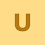### How Accelerometer Works

An accelerometer Sensor is an electromechanical device that measures acceleration forces. These forces may be static or dynamic which is caused by moving or vibrating the accelerometer. By measuring the amount of static acceleration due to gravity, you can find out the angle the device is tilted at with respect to the earth. By sensing the amount of dynamic acceleration, you can analyze the way the device is moving.
There are different types of accelerometers :
• Piezo electric accelerometer
• Piezo resistive accelerometer
• Strain gage accelerometers

How does Piezoelectric accelerometer works
Piezo electric accelerometer is based on principle of piezo electric effect. A Piezo electric substance is one that produces an electric charge when a mechanical stress is applied.  In a Piezo electric accelerometer a mass is attached to a Piezo electric crystal which is in turn mounted to the case of the accelerometer. When the body of the accelerometer is subjected to vibration the mass mounted on the crystal wants to stay still in space due to inertia and so compresses and stretches the piezo electric crystal. This force causes a charge to be generated and due to Newton law (F=ma) this force is in turn proportional to acceleration. The charge output is converted to voltage output by the use of integral electronics (for example: in an IEPE accelerometer) or made available as a charge output (pc /g) in a charge output Piezo electric accelerometer.How does Piezoresistive accelerometer works
A piezo resistive accelerometer is based on piezo resistive effect. The piezoresistive effect describes the changing resistivity of a semiconductor due to applied mechanical stress. The piezoresistive accelerometer uses a piezo resistive substrate in place of the piezo electric crystal and the force exerted by the seismic mass changes the resistance of the etched bridge network and a whetstone bridge network detects this. Piezo-resistive accelerometers have the advantage over piezo-electric accelerometers in that they can measure accelerations down to zero Hertz.• Piezo resistive accelerometers are preferred in high shock applications.
• They can measure accelerations down to zero Hertz.
• Limited high frequency response

How does strain gauge based accelerometer works
A strain gauged accelerometer is based on detecting the deflection of a seismic mass by using a silicon or foil strain gauge element. A whetstone bridge network detects the deflection. The deflection is directly proportional to the acceleration applied to the sensor. Like the piezo-resistive accelerometer it has a frequency response down to zero Hz.• Strain gage accelerometers are preferred in high shock applications.
• They can measure accelerations down to zero Hertz.

• Limited high frequency response

Some of the Key factors while selecting an accelerometer are
Analog vs digital: Depending on the interface to which you will be connecting the accelerometer, you need to select analog or digital output accelerometer.
Output: Accelerometer comes with different outputs-Charge output, IEPE output, Voltage output, current output.
Number of axis: Depending on your requirement you need to select single, double or tri axis accelerometer. The 3 axis accelerometer will measure acceleration in all directions.
Acceleration range:  Acceleration Range is measured in units of g. 1g is equal to the earth's gravity at sea level.
Sensitivity: is the ratio of change in acceleration (input) to change in the output signal. Sensitivity is specified at a particular supply voltage and is typically expressed in units of mV/g.

Applications of Accelerometers:
• Used in cars to study shock and vibrations.
• Camcorders use accelerometers for image stabilization.
• Still cameras use accelerometers for anti-blur capturing.
• Used in mobile phones for multiple functions including tilt detection, motion detection..etc.

1.You are really great because the post you shared here about accelerometer is really interesting and informative too. Thanks for posting this. Keep it up. Thermistors for sale online in USA

2.This is really a very value-able information shared by the author. I really like this blog because when i read this whole blog then i can easily understand the working of Accelerometer sensor . it measures acceleration forces and forces may be static or dynamic which is caused by moving or vibrating the accelerometer.

3.I got some wonderful knowledge from this post. The post is very informative and contains some best knowledge of accelerometer . Thanks for sharing it. watlow pm6